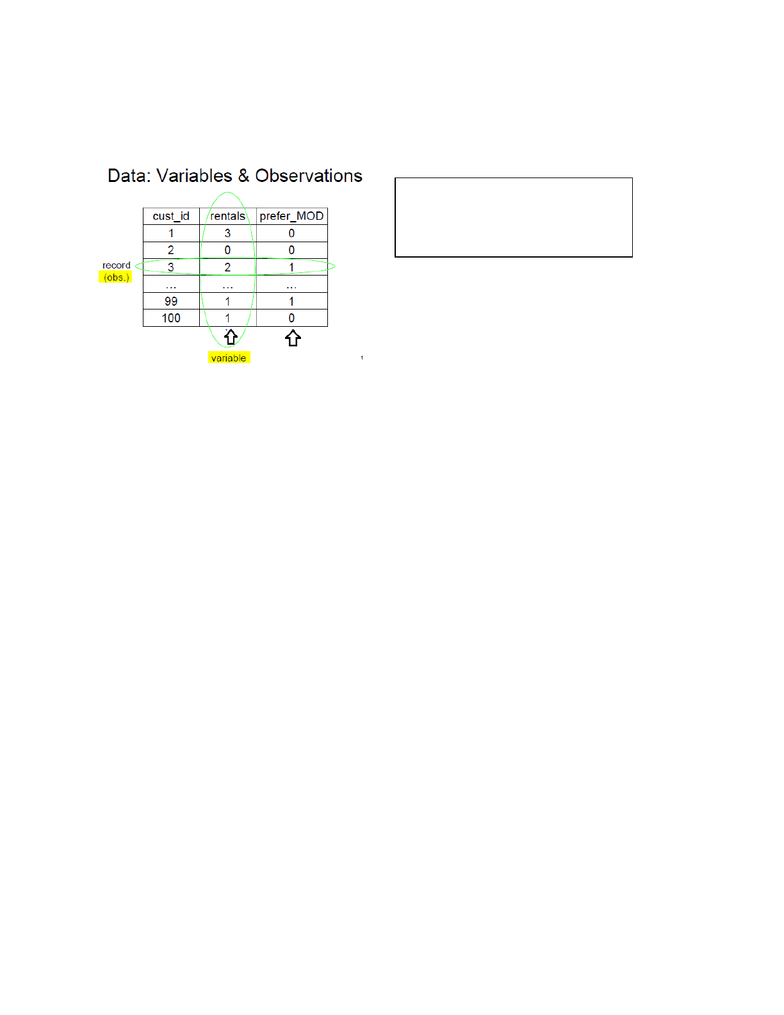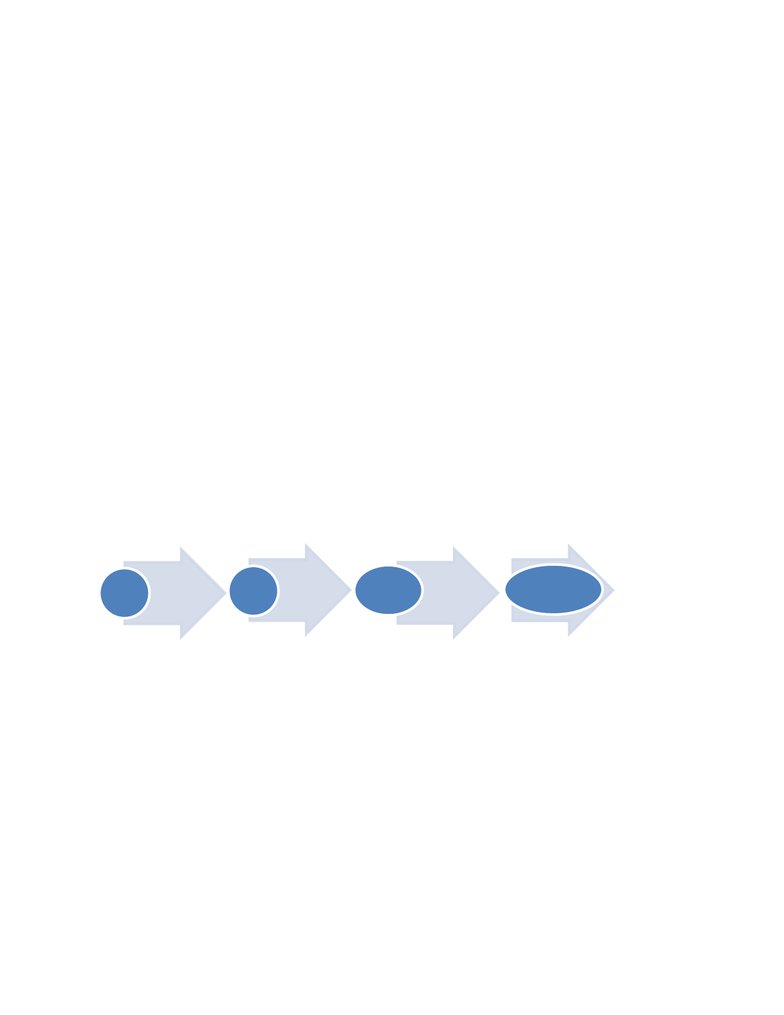# ECO220Y1 Study Guide - Midterm Guide: Mutual Exclusivity, Covariance, Univariate

195 views15 pages
School
Department
CoursePage | 1
ECO220 Notes
Lecture 1: Sampling Errors & Non Sampling Errors
Goal to make inferences about population parameter from sample statistics
Probability: foundation for statistics
Statistics: descriptive and inferential
o Descriptive describes what happened (ex. Class avg)
o Inferential conclusion about data not 100% sure
o Describes sample (data) using statistics
o Make inferences about population and its parameters using observed data (sample)
Population = set of all items of interest (ex. All students @ uoft for evaluations)
Parameter = descriptive measure of a population (something describes
population ex. What %/fraction of population)
Sample = subset of the population (ex. Small group)
Statistics = descriptive measure of a sample (ex. Of 200 in sample response __)
Sampling Error, ‘white noise’, ‘sample noise’, ‘sampling variability = the purely random differences
between a sample and the population that arises b/c the sample is a random subset of the population
As sample size gets larger the sampling error tends to get smaller
Ex. Pick 200 out of 60,000; could result in extremely different (due to change)
o Not wrong b/c random sample nothing wrong with survey itself
Size of sample determines the size of sampling error
o Larger samples = less sampling error
Example: bag of m&m, choose using a spoon, n= # of m&m, y = % of yellow in n
n
y
4
0/4 = 0%
13
2/13 = 15.4%
Population: whole bag of m&m (all)
Parameter: what %/portion are yellow
Sample completed 2 times
Samples statistics %yellow
Law of large Numbers
Larger samples = smaller sampling errors
o Sampling error decrease as n (sample size) increase
o There is no law of small numbers (‘law’ = small samples represent population)
Example: movie on demand, should rural company offer new channel?, randomly select 100ppl, ask 2
different questions
Population: customer base in rural for company
Sample: 100 customers
Sample statistics: mean = 2.3, proportion = 0.45
Population parameters not known b/c sampling errors
The Types of Information
Variables = characteristic recorded about each individual or case (types of info)
Quantitative = numerical measurements of a quality or amount
o Ex. a 10% decrease in prices will lead to a 20% increase in QD
Qualitative = some assessment of quality or kind
o Ex. A increase in price tends to lead to a decrease in QD
Identifier variable = unique code for each product/customer
Unlock document

This preview shows pages 1-3 of the document.
Unlock all 15 pages and 3 million more documents.Page | 2
Data
Rows of data table correspond to individual cases
People answer survey = respondents, people experimented on =
subjects/participants/experimental units
3 Types of Data
Interval = numerical measurements, real numbers that are quantitative/numerical (ex. How
many marriages?)
Ordinal = ranking of categories (ex. How would you rank marital status?)
Nominal = un-ranked categories that are qualitative/categorical (only use names)
Hierarchy of Data
1. Interval - real number -> all calculations are valid
2. Ordinal - must represent the ranked order -> calculations based on ordering process valid
3. Nominal - arbitrary numbers that represents categories -> only calculations based on frequency
3 Types of Data Sets
Cross-sectional = a snapshot of different units taken in the same time period
o Ex. Annual GDP for 2010 for 20 countries (20 observations)
Time Series = track something over time
o Stationary time series = without a strong trend or change in variability (then use
histogram with time series)
o Ex. Annual Canadian GDP from 200 until 2010 ( 10 observations)
Panel (Longitudinal) = a cross-section of units where each is followed over time
o Ex. Annual GDP of 20 countries from 200 unit 2010 (200 observations)
Sampling
Stratified Sampling = a sampling design in which the population is divided into several
homogenous subpopulations, or strata, and random samples are then drawn from each stratum
o Strata = subset of a population that are internally homogenous but may differ from one
another
Systematic Sampling = a sample drawn by selecting individual systematically from a sample
frame
Convenience Sampling = a sampling technique that selects individuals who are conveniently
available
o May not represent population
Cluster sampling = a sample design in which groups, or clusters, representative of the population
are chosen at random and a census is then take of each
Multistage sampling = sampling schemes that combine several sampling methods
# of observations = sample size
‘these data are flawed’
Data = multiple
Datum = 1
Unlock document

This preview shows pages 1-3 of the document.
Unlock all 15 pages and 3 million more documents.Page | 3
Sample size determines what can be concluded from the data regardless of the size of the
population
Voluntary Response Sample
o Hard to define sample frame, doesn’t correspond to population
o Bias toward those with strong opinions (especially negative opinions)
Simple random sample (SRS) = a sample in which each set of n individuals in the population has
an equal chance of selection
Sample Frame = list of individuals from which the sample is drawn
Sampling Vs. Non-sampling Errors
Sampling Error
o Pure chance (random) difference between sample & population (aka ‘white noise’)
o Random: no one can guess the outcome, has some underlying set of outcomes will be
equally likely
o It is impossible to match sample to population b/c too many characteristics to think of
and match
o Undercoverage = not all portions of population sampled
Non-sampling Errors
o Systematic (not random) difference between sample & population
o Biased estimate = statistic is systematically higher or lower than the parameter
o Systematic errors in data collection:
Systematic lying (ex. Ppl over estimate income)
Poor survey instrument design (ex. Unclear)
o Non-response bias:
Low response rate and non-responders are non-random (ex. Selection)
o Sampling frame differs from target population
o Sampling variability = the sample-to-sample differences
Population Parameter = a numerically valued attribute of a model for a population (hope to estimate
from sample data)
Biased = any systematic failure of sampling method to represent its population
Measurement error = intentional or unintentional inaccurate response to a survey question
Valid Survey
Know what you want to know
Use the right sampling frame
Ask Specific rather than general question
Watch for biases
o Nonresponsive bias = bias introduced to a sample when a large fraction of those
sampled fails to respond
o Voluntary Response Bias
o Response Bias = tendency of respondents to tailor their responses to please interviewer
and consequence of slanted question wording
Be careful with question phrasing
used to
calculate
Sample used to
estimate
Statistic Tells us
Parameter Population
Unlock document

This preview shows pages 1-3 of the document.
Unlock all 15 pages and 3 million more documents.